Finding principal value

Chapter 2 Class 12 Inverse Trigonometric Functions
Concept wise

Suppose we are given

cos -1 (1/2)

Principal value of cos -1 (1/2) means

the angle x where cos x  = 1/2

We use this table for our help

 Range Positive Negative sin -1 [-π/2, π/2] θ – θ cos -1 [0,π] θ π – θ tan -1 (-π/2, π/2) θ – θ

1. Put y = cos -1 (1/2)
2. So, cos y = 1/2
3. Now ignore the signs and find value of θ
4. Using the table, Find principal value according to the table

Let's look at some examples

## Find the principal value of cos –1 ( 1/2 )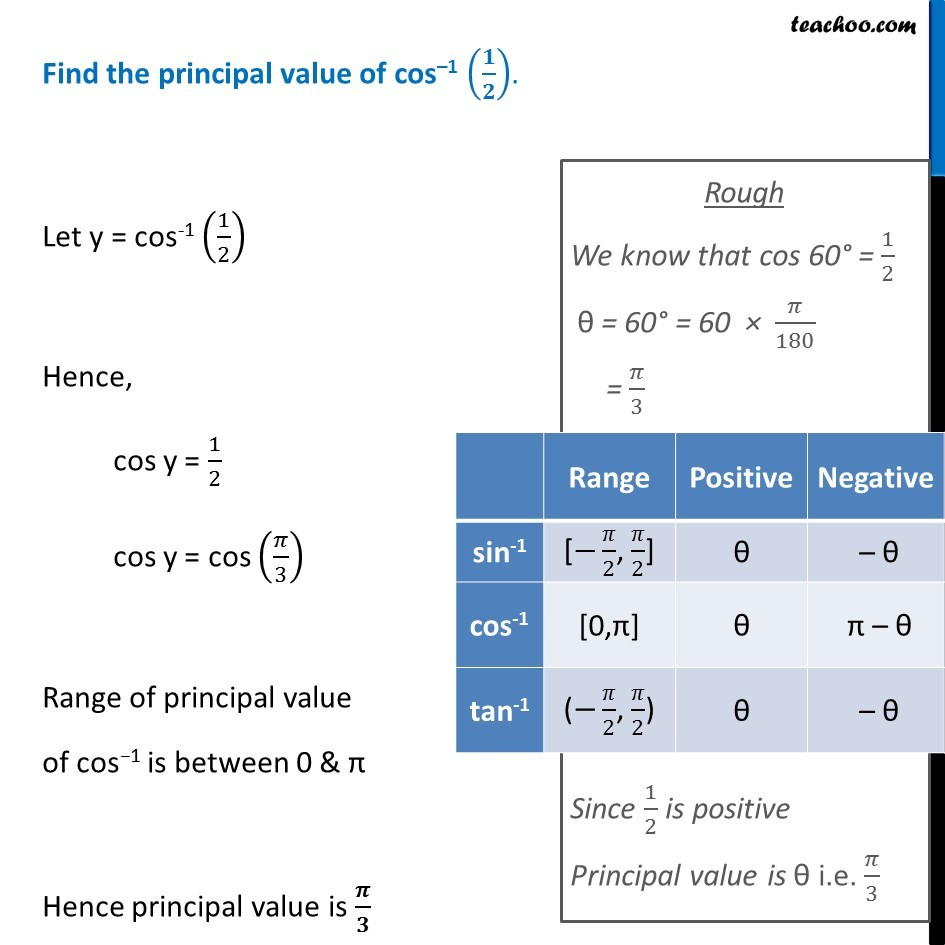## Find the principal value of cos –1 (-1 /2 )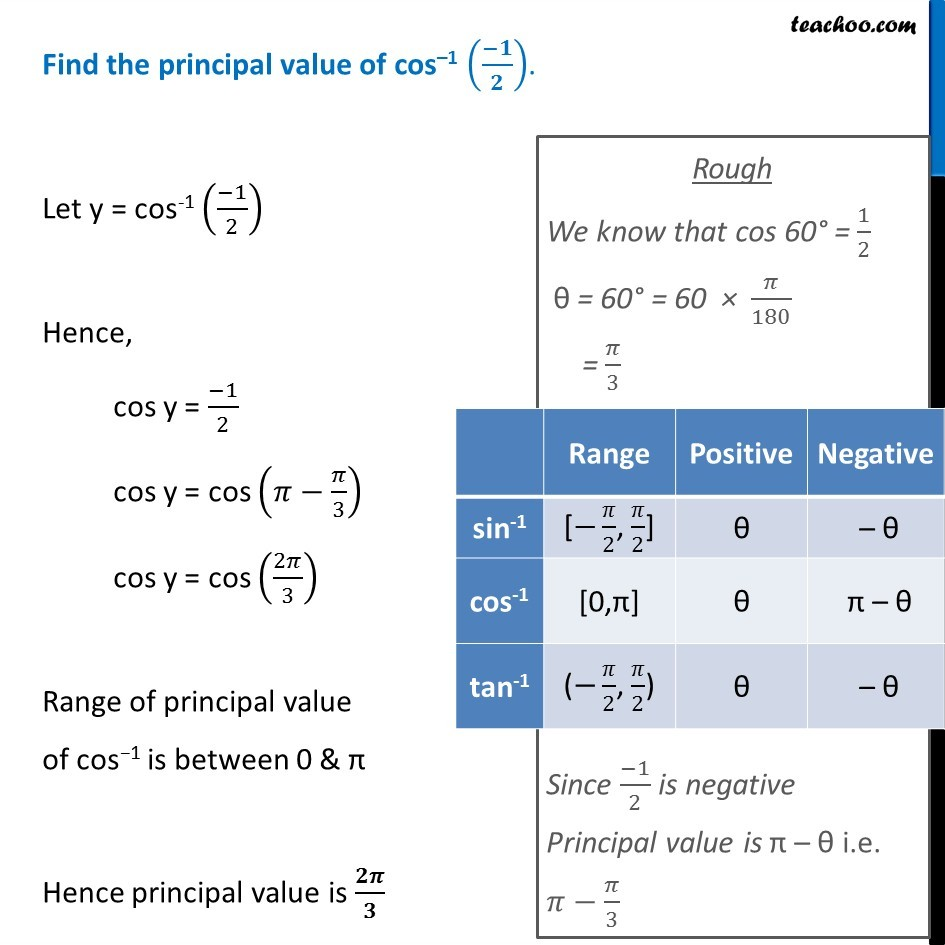## Find the principal value of sin –1 ( 1/2 )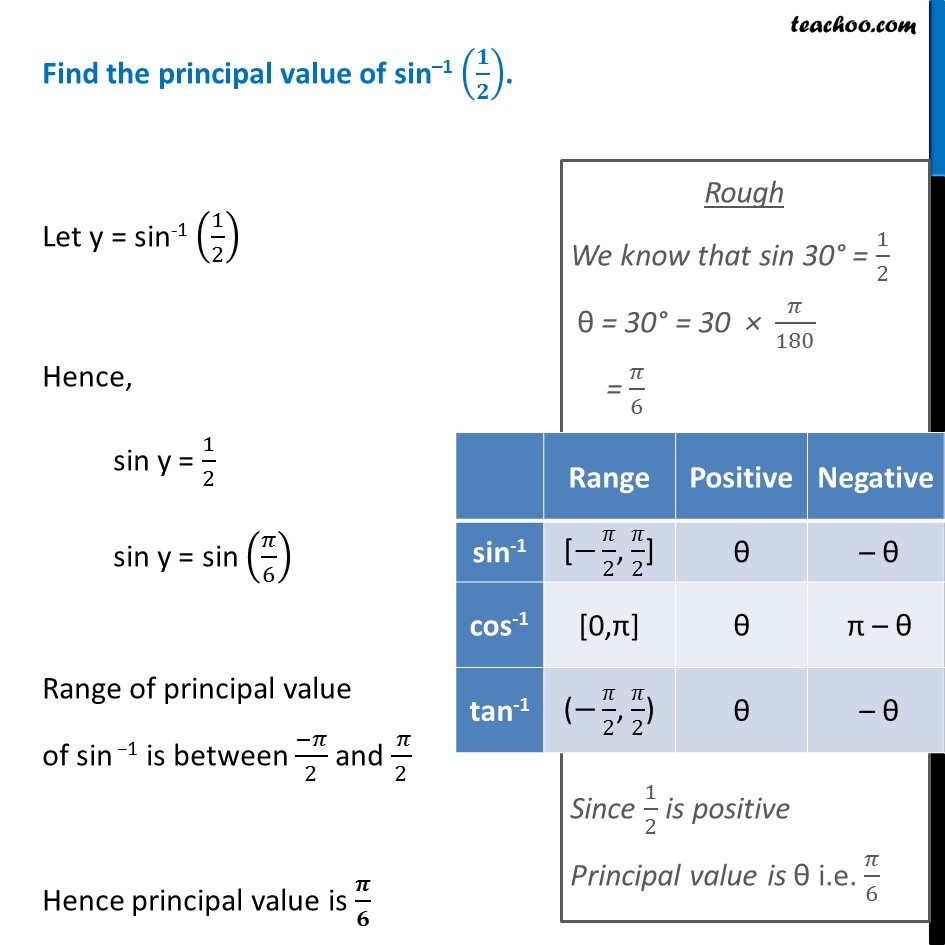## Find the principal value of sin –1 (-1/2)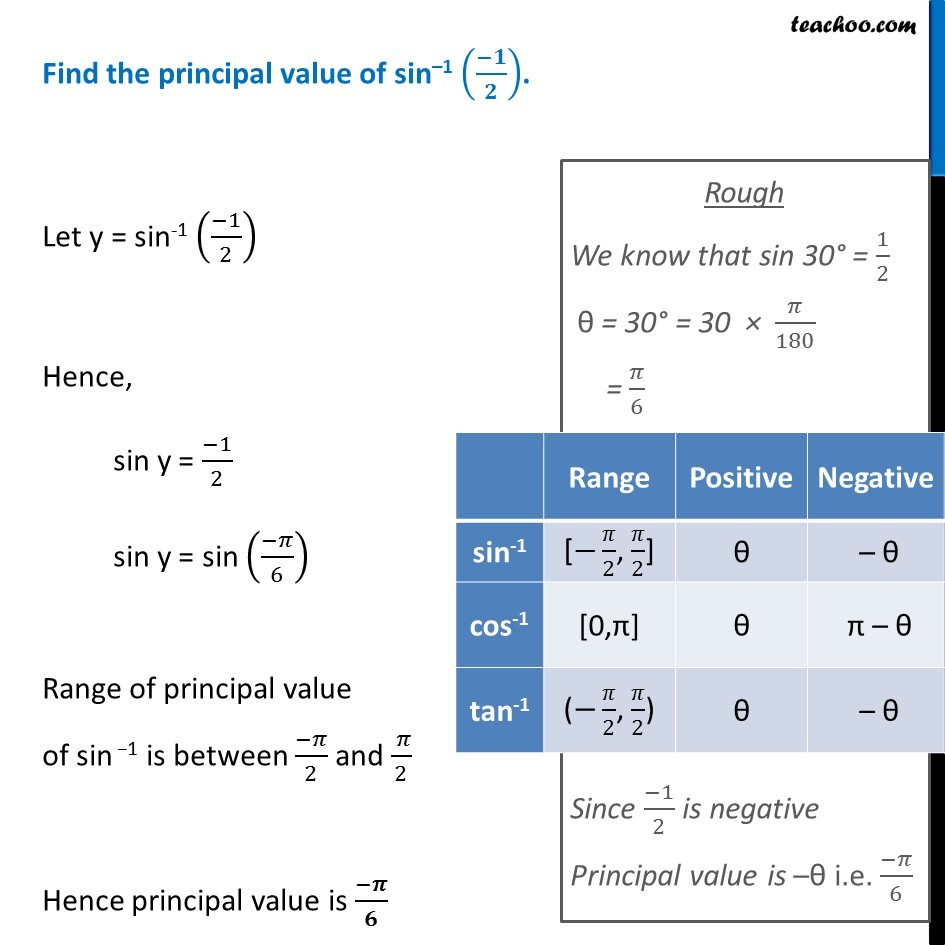## Find the principal value of tan –1 (1)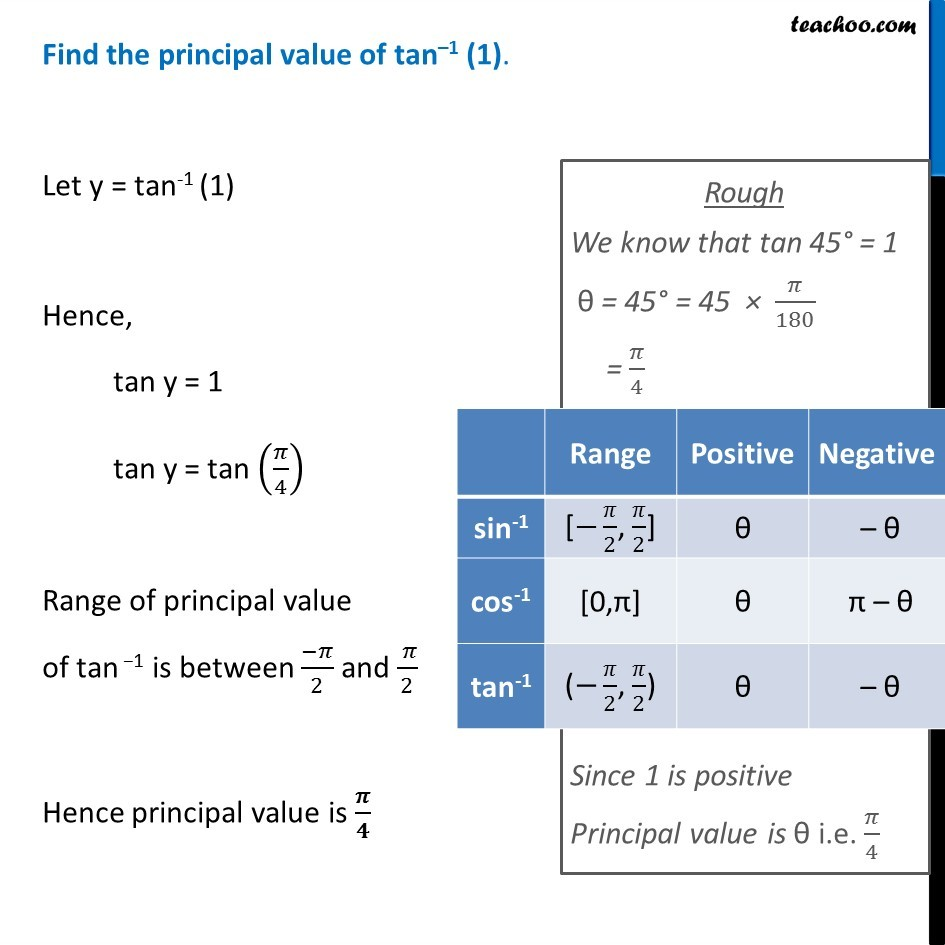## Find the principal value of tan –1 (-1 )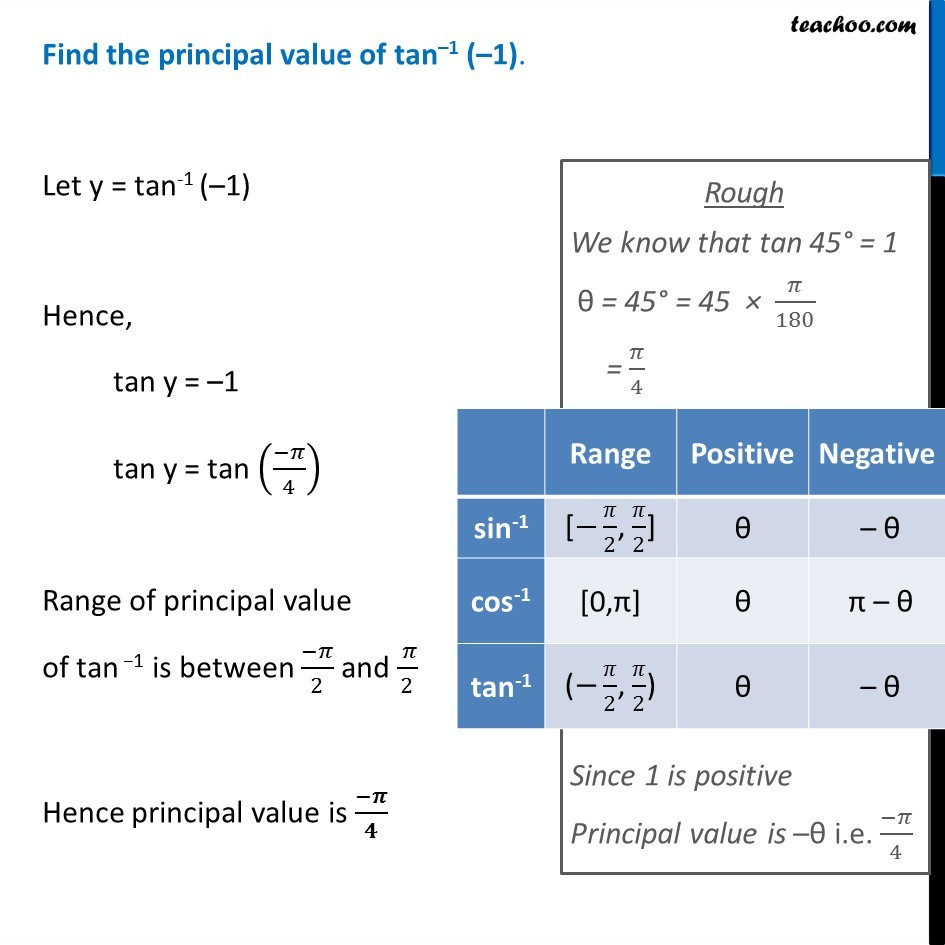Learn in your speed, with individual attention - Teachoo Maths 1-on-1 Class

### Transcript

Find the principal value of cos–1 (𝟏/𝟐). Let y = cos-1 (1/2) Hence, cos y = 1/2 cos y = cos (𝜋/3) Range of principal value of cos−1 is between 0 & π Hence principal value is 𝝅/𝟑 Rough We know that cos 60° = 1/2 θ = 60° = 60 × 𝜋/180 = 𝜋/3 Since 1/2 is positive Principal value is θ i.e. 𝜋/3 Find the principal value of cos–1 ((−𝟏)/𝟐). Let y = cos-1 ((−1)/2) Hence, cos y = (−1)/2 cos y = cos (𝜋−𝜋/3) cos y = cos (2𝜋/3) Range of principal value of cos−1 is between 0 & π Hence principal value is 𝟐𝝅/𝟑 Rough We know that cos 60° = 1/2 θ = 60° = 60 × 𝜋/180 = 𝜋/3 Since (−1)/2 is negative Principal value is π – θ i.e. 𝜋−𝜋/3 Find the principal value of sin–1 (𝟏/𝟐). Let y = sin-1 (1/2) Hence, sin y = 1/2 sin y = sin (𝜋/6) Range of principal value of sin −1 is between (−𝜋)/2 and ( 𝜋)/2 Hence principal value is 𝝅/𝟔 Rough We know that sin 30° = 1/2 θ = 30° = 30 × 𝜋/180 = 𝜋/6 Since 1/2 is positive Principal value is θ i.e. 𝜋/6 Find the principal value of sin–1 ((−𝟏)/𝟐). Let y = sin-1 ((−1)/2) Hence, sin y = (−1)/2 sin y = sin ((−𝜋)/6) Range of principal value of sin −1 is between (−𝜋)/2 and ( 𝜋)/2 Hence principal value is (−𝝅)/𝟔 Rough We know that sin 30° = 1/2 θ = 30° = 30 × 𝜋/180 = 𝜋/6 Since (−1)/2 is negative Principal value is –θ i.e. (−𝜋)/6 Find the principal value of tan–1 (1). Let y = tan-1 (1) Hence, tan y = 1 tan y = tan (𝜋/4) Range of principal value of tan −1 is between (−𝜋)/2 and ( 𝜋)/2 Hence principal value is 𝝅/𝟒 Rough We know that tan 45° = 1 θ = 45° = 45 × 𝜋/180 = 𝜋/4 Since 1 is positive Principal value is θ i.e. 𝜋/4 Find the principal value of tan–1 (–1). Let y = tan-1 (–1) Hence, tan y = –1 tan y = tan ((−𝜋)/4) Range of principal value of tan −1 is between (−𝜋)/2 and ( 𝜋)/2 Hence principal value is (−𝝅)/𝟒 Rough We know that tan 45° = 1 θ = 45° = 45 × 𝜋/180 = 𝜋/4 Since 1 is positive Principal value is –θ i.e. (−𝜋)/4